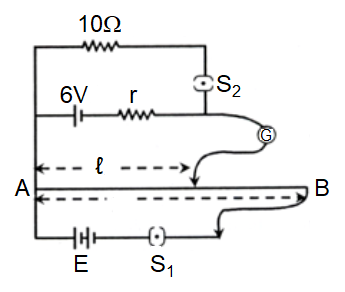potentiometer
Question

# In the arrangement shown in the figure when the switch is open, the galvanometer shows no deflection for l = L/2. When the switch  is closed, the galvanometer shows no deflection for l = $\frac{5}{12}\mathrm{L}$ . The internal resistance (r) of 6 V cell, and the emf E of the other battery are respectivelyModerate
Solution

## When ${\mathrm{S}}_{2}$ is open, l= L/2 $\therefore \mathrm{E}=\left(\frac{6\mathrm{V}}{\mathrm{L}/2}\right)\mathrm{L}=12 \mathrm{V}$when ${S}_{2}$ is closed ${\mathrm{V}}_{10}=\left(\frac{12}{\mathrm{L}}\right)×\frac{5}{12}\mathrm{L}=5$$10×\left(\frac{6}{10+\mathrm{r}}\right)=5 ⇒ \mathrm{r}=2 \mathrm{\Omega }$

Get Instant Solutions